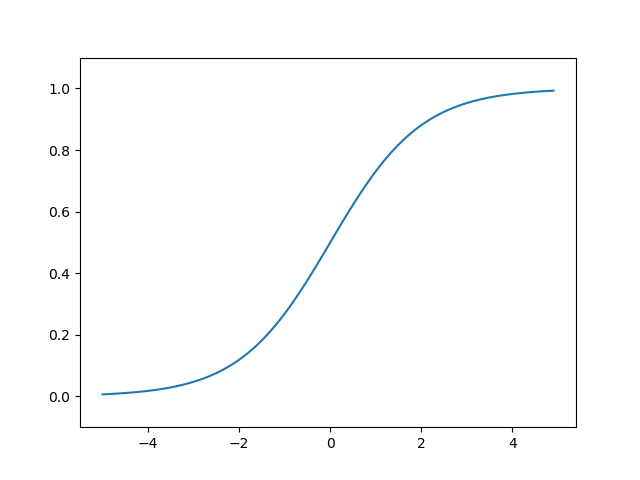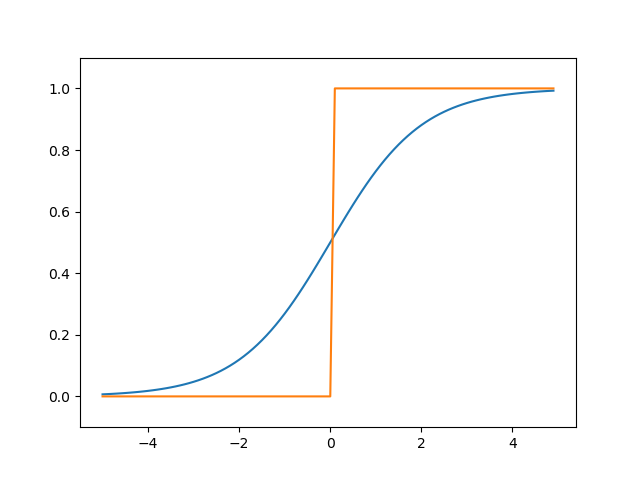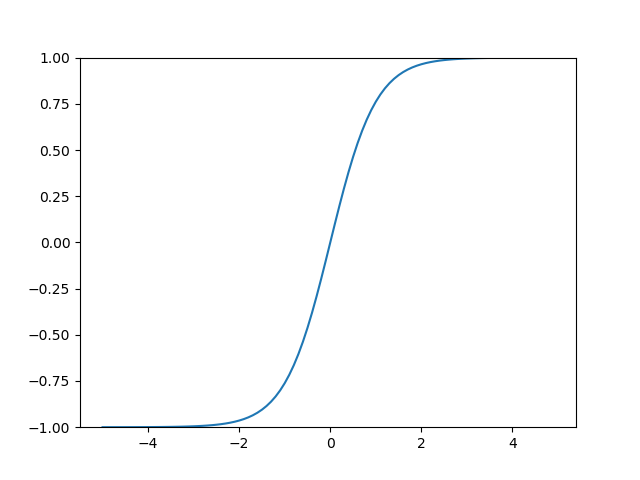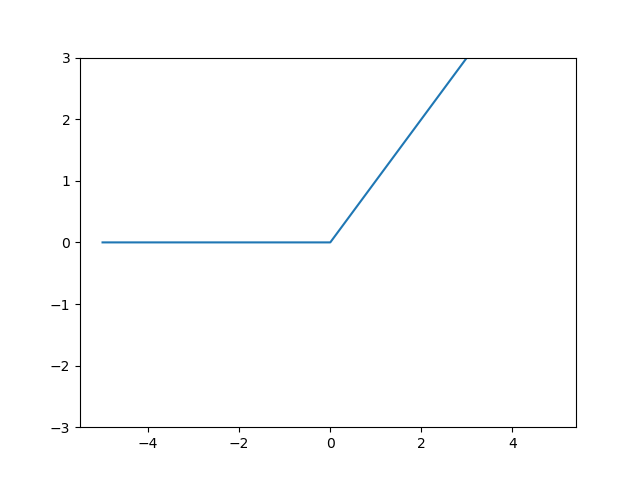## 阶跃函数

 1 2 3 4 5  def step_function(x): if x > 0: return 1 else: return 0 

 1 2 3 4 5 6 7  import numpy as np def step_function(x): y = x>0 return y.astype(np.int) print(step_function(np.array([1.0,2.0,0,-2.3]))) 

y=x>0 利用numpy的广播功能，将numpy数组依次和0进行对比，当大于0的时候返回True，否则返回False,再将True和False转换为int的0和1

 1 2 3 4 5 6 7  >>> import numpy as np >>> x = np.array([1.0,2.0,0,-2.3]) >>> y = x>0 >>> y array([ True, True, False, False]) >>> y.astype(np.int) array([1, 1, 0, 0]) 

  1 2 3 4 5 6 7 8 9 10 11 12 13 14  #coding:utf-8 import numpy as np import matplotlib.pylab as plt def step_function(x): y = x>0 return y.astype(np.int) x = np.arange(-5.0, 5.0, 0.1) y = step_function(x) plt.plot(x, y) plt.ylim(-0.1, 1.1) # 指定y轴的范围 plt.show() 

## sigmoid函数

sigmoid函数的数学表达式为python 的函数表达为

 1 2 3 4 5 6 7 8  #coding:utf-8 import numpy as np def sigmoid(x): return 1 / (1 + np.exp(-x)) print(sigmoid(np.array([-2.0,1.0,2.0]))) 

sigmoid的函数图形为1 2 3 4 5 6 7 8 9 10 11 12 13 14 15 16 17 18 19 20 21 22  #coding:utf-8 import numpy as np import matplotlib.pylab as plt def step_function(x): y = x>0 return y.astype(np.int) def sigmoid(x): return 1 / (1 + np.exp(-x)) print(sigmoid(np.array([-2.0,1.0,2.0]))) x = np.arange(-5.0, 5.0, 0.1) y = sigmoid(x) ys = step_function(x) plt.plot(x, y) plt.plot(x, ys) plt.ylim(-0.1, 1.1) # 指定y轴的范围 plt.show()## tanh函数

tanh的数学表达式为python函数表达为

 1 2 3 4  def tanh(x): return np.true_divide(np.exp(x) - np.exp(-x),np.exp(x) + np.exp(-x)) print(tanh(np.array([-11,-10,-9,-2.0,0,2.0,3.0,10.0,11.2]))) 

 1 2  [-1. -1. -0.99999997 -0.96402758 0. 0.96402758 0.99505475 1. 1. ] 

tanh的图形为## ReLU函数

ReLU函数在输入大于0 时，直接输出该值；在输入小于等于0 时，输出0，数学表达式为ReLU的python表达式为

 1 2 3 4  def relu(x): return np.maximum(0,x) print(relu(np.array([-2.0,1.0,2.0]))) 

ReLU的图形为